Next: Bibliography

The Physical Basis of the Direction of Time
by H. D. Zeh

Book Review by John C. Baez

April 6, 1993

This appeared in the Mathematical Intelligencer.
Also available in PDF, Postscript and LaTeX.

In this book Zeh brings some clarity to a very murky problem: why are the future and the past so different? One need only to read the physics journals to see that this is a multi-faceted and very real issue that vexes the experts even now. To understand its seriousness, it is first necessary to see how similar the future and the past are. They don't seem so in everyday life: we remember the past but not the future, our actions affect the future but not the past, and so on. From this standpoint it is really quite surprising that the dynamical laws of physics — with one small exception — seem to be symmetrical under time reversal.

Before we go any further, it's important to get a clear idea of what time reversal symmetry really means. At the simplest level, we may think of the laws of physics as equations involving a time variable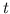, and say that they are symmetric under time reversal if given any solution, and making the substitution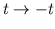, we obtain another solution. To take an easy example, consider a point particle of mass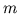in three-dimensional space with no forces acting on it. If we write its position as a function of time,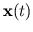, Newton's second law says that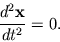Ifsatisfies this equation then so does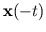.

Typically the laws are more complicated, and one may have to be more careful in defining time reversal symmetry. Different laws of physics involve very different mathematical structures, but they are almost always separated into two components, the "kinematics'' and the "dynamics''. The kinematics consists of the description of the set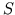of states that the system can be in at any given time. For example, in classical mechanics, we can specify the state of a point particle in three-dimensional space by giving its positionand its velocity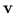, so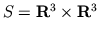. The dynamics tells how states change with time. In a theory where we can predict both the future and past from the present, and where there are no time-dependent external influences, we usually describe dynamics with a family of maps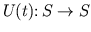, where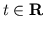. If the state of the system is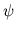at some time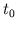, the state is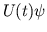at time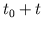. The maps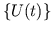should form a "one-parameter group,'' that is,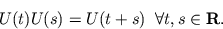We say that the physical system given by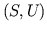has "time-reversal symmetry'' if there is a map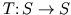, called time reversal, such that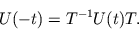For example, our point particle with no forces on it moves with constant velocity, so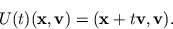It's easy to check that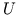is a one-parameter group, and that the system has time-reversal symmetry, whereNote, by the way, that time-reversal symmetry in the sense described above is different from requiring that a given statebe invariant under time-reversal: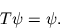Our world is evidently in a state that is not even approximately invariant under time reversal; there are many processes going on whose time-reversed versions never seem to happen. But this is logically independent from the question of whether the dynamical laws of physics admit time reversal symmetry. Keeping this distinction straight is crucial for thinking clearly about the direction of time. Even people who claim to understand the distinction often slip. When reading about time reversal symmetry, I become infuriated when authors confuse symmetry of the laws with symmetry of the state, and I am happy to report that not once did I hurl Zeh's book to the floor in anger.

At this point, we could go through all theories of physics and check to see whether they have time reversal symmetry. But let us simply turn to the most up-to-date and complete laws of physics we know: the standard model and general relativity. The "standard model'' is a complicated theory of quantum fields that describes the most fundamental particles we know (mainly leptons and quarks) and the forces — electromagnetism and the weak and strong nuclear forces — by which they interact. In other words, it treats everything except gravity. The standard model has time-reversal symmetry except for effects involving the weak force. This is the force that permits a proton and electron to turn into a neutron and a neutrino, as happens in some radioactive atoms, or vice-versa, as in some others.

In fact, in quantum field theory, time reversal, or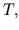is one of a trio of possible symmetries, the others being charge conjugation or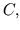which amounts to interchanging particles with their antiparticles, and parity, or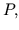which is related to spatial inversion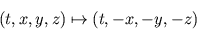in somewhat the same way that time reversal relates to the map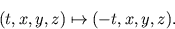In a quantum field theory states are given by unit vectors in a Hilbert space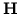. The symmetries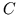and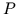are given by unitary operators on— if the theory in question admits these symmetries — while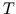is given by an antiunitary operator, that is, a conjugate-linear one-to-one and onto norm-preserving map fromto itself. I will restrain myself from explaining whymust be antiunitary rather than unitary, fascinating though this is. The key point here is that in the standard model, the weak force violates,, andsymmetry, while electromagnetism and the strong force admit all these symmetries. Moreover, while violation ofsymmetry is quite common and blatant - particularly for neutrinos — violation ofsymmetry has so far only been seen in the decays of a single particle, the neutral kaon, and the amount of violation is minute. Most physicists believe that this smallsymmetry violation is not particularly related to the gross time asymmetry of the state of the universe. But there is something very curious about this, as in the elaborate Islamic designs that are perfectly symmetrical except for one tiny flaw put in to avoid the wrath of Allah. Zeh's book does not treat theasymmetry of the weak interaction very thoroughly, but luckily there is already a good book that does just this .

On the other hand, general relativity treats gravity, which is a great puzzle in its own right, since it seems very difficult to unify with the rest of the forces. Unlike all the other forces, it is not at all natural to formulate its dynamics in terms of a one-parameter time evolution group. Essentially, this is because it treats of the geometry of spacetime itself, and how it wiggles around. While the dynamics of general relativity is by now moderately well understood, the modifications required for a quantum theory of gravity are still very poorly understood, and seem to require a radical rethinking of the very notion of time. In his last chapter, "The Quantization of Time,'' Zeh tours this fascinating subject. While a quantum theory of gravity would be likely to have profound implications for the study of time reversal, one can fairly say that so far the dynamics of gravity seems to admit time reversal symmetry.

It's worth noting that there are some cases where at first glance it looks as if the laws of physics are asymmetric under time reversal, but on closer inspection it turns out to be the fault of the particular state of the universe we are in. The two most famous examples are the "time arrow of radiation'' and the "time arrow of thermodynamics.'' Here an "arrow of time'' is used loosely to denote something that is not symmetric under time reversal.

The time arrow of radiation refers simply to the fact that when we shake an electrically charged object, it emits waves of radiation that ripple outwards as time progresses into the future, rather than the past. This is expressed mathematically in terms of what are called Green's functions. To understand these, it's easier to consider the scalar wave equation rather than Maxwell's equations of electromagnetism in their full glory. Thus we have a "field''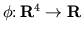being produced by a "source''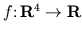, and we assume both are smooth functions and that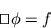where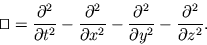The source does not uniquely determine the field, but it is possible to write down formulas that give us for any source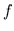a field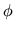with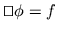. In particular, we say that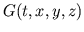is a Green's function (actually a distribution) for the scalar wave equation if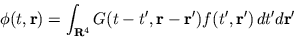where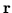is short for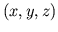, implies that. Two Green's functions are the "advanced'' one,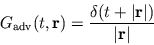and the "retarded'' one,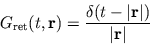where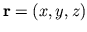and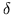is the Dirac delta distribution. In electromagnetism one typically uses the retarded Green's function, so that if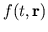is nonzero only for times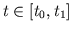, thenis typically nonzero after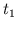, but is zero before.

It may seem odd that while the equationis preserved by the transformation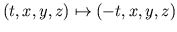, we are solving it in a way that doesn't respect this symmetry. But there are two things that help resolve this puzzle. First, it is worth noting that working with the retarded rather than the advanced Green's function is, at least forvanishing outside a bounded set, equivalent to an assumption about the nature of the field, namely that it vanish as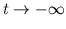. In short, we are making a time-asymmetric assumption about the state of the system when we are choosing the retarded Green's function. Why do we make this assumption? For a quite interesting reason: because it's dark at night. In a sense, light radiates out from the sun and from our flashlights, rather than coming into them from the distance, because the universe is a rather dark and cold place. The very fact that space is mostly dark and empty, with a speckling of hot bright stars that radiate outwards, is blatantly time-asymmetric, so the time arrow of radiation appears to be cosmological in origin. This fact about the universe is crucial to life as we know it, since all life on earth is powered by the outgoing radiation of the sun, and the earth in turn dumps its waste heat into the blackness of space.

A second, subtler point is that the equationdoes not fit into the general framework of one-parameter groups, because the fieldis subject to an arbitrary time-dependent external influence, the source. Here one wants to imagine oneself, the experimenter, as being able to do whatever one wants with the source, and see what it does to the field. This is related to the notion of free will: we like to think that the laws of physics govern the behavior of everything else, but that we are free to do whatever we want. However, in the most fundamental laws of physics we know - the standard model and general relativity — no "arbitrary external influences'' appear. In these laws, there is no need to choose between a retarded and advanced Green's function (or some other Green's function, for that matter). There is only the need to choose the state that best matches what we observe.

The time arrow of thermodynamics is perhaps the most famous aspect of time reversal symmetry — so I will treat it very briefly here. Why is it so much more likely that a porcelain cup will fall to the floor and smash to smithereens, than it is for a pile of porcelain smithereens to form into a cup and jump into ones hand? Disorder seems to be always on the increase. In fact, in thermodynamics there is a quantity called entropy,, which is a a measure of disorder — although one must be very careful not to fall for the negative connotations of "disorder,'' which here is interpreted in a very precise and sometimes counterintuitive sense. The second law of thermodynamics is that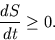This law appears utterly time-asymmetric, and reconciling it with the (almost) time-symmetric fundamental laws of physics has exercised the minds of many physicists for many years. But the final resolution seems to be a simple one: this law is not true except for certain states. That is, it expresses a time asymmetry of the state of a system, rather than an asymmetry in the dynamical laws. As long as the dynamical laws admit symmetry under time reversal, for every state with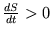there is a time-reversed state with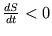. It is also worth noting that the vast majority of states typically havequite large and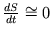.

As with the time arrow of radiation, in the last analysis it appears to be nothing but a raw experimental fact that the entropy of our universe is increasing. In a sense this is not surprising, because pondering chemistry and biology a bit it becomes apparent that life as we know it requires the entropy to be changing monotonically, rather than staying about the same. One might ask whyrather than, but this is essentially a matter of convention. Processes like remembering and planning, which define the psychological notions of future and past, are only able to occur in the direction of increasing entropy. That is, a memory at timecan only be of an event at time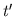for which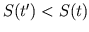, while a plan at timecan only be for an action at timefor which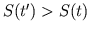. Since we have settled on using calendars for which the number of the years increase in the direction of plans, rather than memories, we have chosen a time coordinatefor which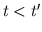implies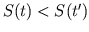.

The main remaining mystery, then, is why the state of the universe is grossly asymmetric under time reversal, even though the dynamical laws of physics are almost — but not quite! — symmetric. If the reader wishes to puzzle over this some more, or wants supporting evidence for some of the (perhaps upsetting) claims I've made above, he or she could not do better than to read Zeh's book.